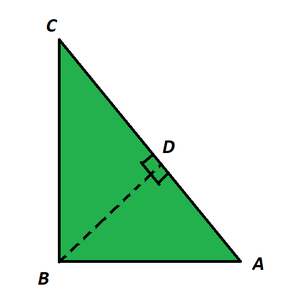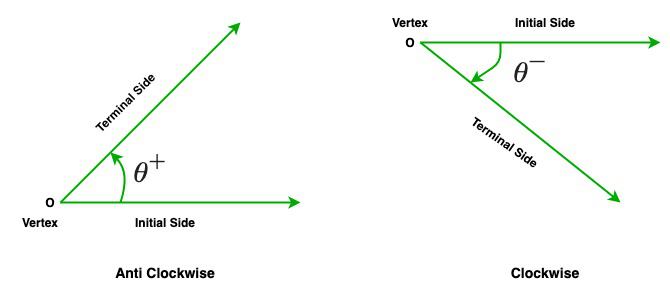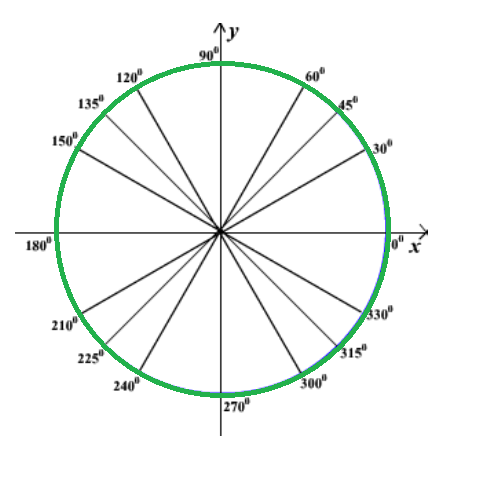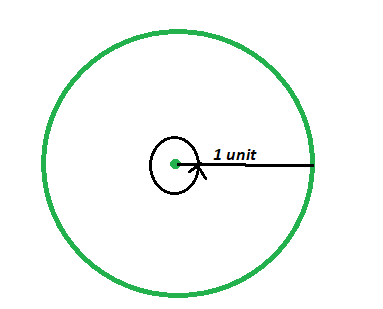Open in App
Not now

# Measurement of Angles

• Last Updated : 15 Jun, 2022

In mathematic, the word ‘trigonometry’ is obtained from the two Greek words where ‘trigon’ and ‘metron’ which means ‘measuring the sides of a triangle. So, the trigonometric functions express the relationship between an angle of a right-angled triangle and the ratios of its two sides, trigonometric functions are also known as angle functions. Generally, sine, cosine, and tangent are used in modern mathematics as compared to cosecant, the secant, and the cotangent. Now each of these six trigonometric functions has corresponding inverse function known as inverse trigonometric function.

There are a number of trigonometric equations and identities that denote the relationship between the functions and help to find the angles. In this article, we will study the concept of a triangle, trigonometric ratios, and functions along with various angles and measurement degrees.

### Basic concept of triangle

Properties of Triangle:

• A triangle has 3 sides, 3 vertices, and 3 angles.
• The sum of all the interior angles of a triangle is always equal to 180°, it is known as the angle sum property of a triangle.
• The difference between the length of any two sides is always lesser than the third side.
• The area of the triangle is 1/2 × base × height

Angles of the triangle:

• A right-angled triangle: It has one right angle. A right angle is an angle of size 90°.
• An obtuse triangle: It has one obtuse angle. An obtuse angle is an angle of size greater than 90° but less than 180°.
• An acute triangle: It has acute angles which is less than  90° but greater than 0°.

Pythagoras Theorem:

In a right-angled triangle, the sum of the squares of the two sides(other than hypotenuse) of a  right-angled triangle is equal to the square of the hypotenuse side. Or in other words, the hypotenuse is the longest side of a right-angled triangle and it is opposite to the angle of 90°.

Formula:

hypotenuse2 = perpendicular2+ base2

Or c2 = a2 + b2

Where a is the perpendicular side, b is base, and c is the hypotenuse side

Note: Pythagoras theorem is only applicable to Right-Angled triangles.

Proof Of Pythagoras Theorem:

Given: A right-angled triangle ABC, whose right angle is at B.

To Prove: AC2 = AB2 + BC2

Construction: Construct a line from angle B to the line AC such that it makes a angle of 90° with AC.Proof:

As we know that, △ADB ~ △ABC

Therefore, AD/AB = AB/AC (corresponding sides of similar triangles)

Or, AB2 = AD × AC ……………(1)

Also, △BDC ~△ABC

Therefore, CD/BC = BC/AC (corresponding sides of similar triangles)

Or, BC2 = CD × AC ……………(2)

On adding the equations (1) and (2) we get,

AB2 + BC2 = AD × AC + CD × AC

AB2 + BC2 = AC (AD + CD)

Since, AD + CD = AC

So, AC2 = AB2 + BC2

Hence proved

### Trigonometric Ratios

In trigonometry, there are 6 ratios that are used for finding the angles, they are known as trigonometric functions. And these six trigonometric functions are sine, cosine, secant, co-secant, tangent, and co-tangent.

The trigonometric functions are extracted by using the Pythagoras theorem and right-angle triangles. The trigonometric ratios are:

sin θ = P/H

cos θ = B/H

tan θ = P/B = sin θ/cos θ

cot θ = 1/tan θ = cos θ/sin θ = B/P

cosec θ = 1/sin θ = H/P

sec θ = 1/cos θ = H/B

Trigonometric Table: The trigonometric table is basically a systematic collection of values of trigonometric functions (sin, cos, tan, cot, sec and cosec) on standard angles including 0°, 30°, 45°, 60°, 90°. However, other angles can also be found by using this table.

Trigonometric Identities:

• Pythagorean Identities:

Pythagorean identity are those identities which are used in showing the Pythagorean theorem in the terms of trigonometric functions.

sin 2θ + cos2 θ = 1

1 + tan2 θ = sin2 θ

1 + cot2 θ = cosec2 θ

• Reciprocal Identities

cosec θ = 1/sin θ

sec θ = 1/cos θ

cot θ = 1/tan θ

sin θ = 1/cosec θ

cos θ = 1/sec θ

tan θ = 1/cot θ

• Co-function Identities:

The cofunction identities indicates the relationship between sin, cos, tan, cot, sec and cosec. The value of a trigonometric function of an angle is equal to the value of the cofunction of the complement. You should remember this ‘that a complement is defined as two angles whose sum is 90°’.

sin(90 − θ) = cos θ

cos(90 − θ) = sin θ

tan(90 − θ) = cot θ

cot(90 − θ) = tan θ

sec(90 − θ) = cosec θ

cosec(90 − θ) = sec θ​

• Sum and Difference Identities:

sin(x + y) = sinx cosy + cosx siny

cos(x + y) = cosx cosy – sinx siny

tan(x + y) =sin(x – y) = sinx cosy – cosx siny

cos (x – y) = cosx cosy + sinx siny

tan (x − y) =cot (x + y) =cot (x – y) =• Double Angle Identities:

Double, means when the size of the angle gets double of the previous.

sin(2x) = 2 sinx cosx =cos(2x) = cos2x – sin2x =cos(2x) = 2 cos2x − 1 = 1 – 2 sin2x

tan(2x) =sec (2x) =• Triple Angle Identities:

sin 3x = 3 sinx – 4 sin3x

cos 3x = 4 cos3x-3 cos x

tan 3x =• Sum to Product Identities:

sinx + siny =sinx – siny =cosx + cosy =cosx – cosy =### Angle Measurement

Angle is a measure of rotation of a given ray from its initial point. The original ray is known as the initial side of the angle and the final position of the ray after rotation is known as the terminal side. The point of rotation is known as the vertex. If the direction of rotation is anticlockwise, then the angle is said to be ‘+’ve and if the direction of rotation is clockwise, then the angle is ‘-‘ve. In trigonometry, the angle value ranges from 0-360.There are two units of measure for angles namely:

1. degrees

Degree Measurement:

In general, we can measure an angle by determining the amount of rotation it takes from the initial side to the terminal side. So, we can measure an angle using degrees. A measure of one degree (1°) is equivalent to a rotation of 1/360 of a complete revolution. Here, a revolution is used to measure an angle which is created when the initial side rotates all the way around its vertex until it reaches again its initial position.

When we measure an angle, it is convenient to mark degrees on the circumference of a circle. Hence, on a complete revolution then the angle is 360°, on half a revolution then the angle is 180°, on a quarter of a revolution then the angle is 90°, and so on.

one complete angle = 360 °

one degree = rotation of (1/360)th of a complete revolution

1° = 60 minutes

1° = 60′

1 minute = 60 seconds

1′ = 60”We can also measure an angle using radian. A radian measure is the ratio of the length of a circular arc to the radius of the arc. Since radian it is the ratio of a length to the length, a result is a pure number that does not need any unit symbol.

1 radian = Angle subtended by an arc of unit length at the centre point of the circle.

1 unit length of arc = 1 radian

2 unit length of arc = 2 radian

2π unit length of arc (complete revolution) = 2π radian

1 complete revolution = 360° = 2πc

In a circle, if the radius of the circle is r, a arc length l subtends an angle θ at the center, then θ (in radian) = l/r or l = rθ. Where l = length of arc and, r = radius of the circle.As we know that a circle subtends at its centre an angle whose measure is 2π radians as well as 360°.

As we know that π = 22/7

so 1 radian = 180°/π = 57°16′(approx)

also, 1° = π /180 = 0.0174(approx)

Formula:

Angle in Radian = Angle in Degree x π/180

or

Angle in Degree = Angle in Radian x π/180

The below table shows the relationship between degree, radian, and revolution:

### Sample Problems

Question 1. Convert 90 degrees to radians.

Solution:

Given, 90° i.e. the angle

As we know that,

Angle in radian = Angle in degree x (π/180)

= 90 x (π/180)

= π/2

Hence, 90 ° is equal to π/2 in radian.

Question 2. Convert π/6 into degrees.

Solution:

By Using the above formula

we get,  π/6 × (180/π)

= 180/6

= 30 °

Question 3. Convert 15 degrees to radian.

Solution:

By Using the above formula,

we get, 15 x π/180

= π/12

Question 4. If cos x = -4/5 and x lies in the third quadrant, the find the value of sin x, tan x.

Solution:

Given that cos x = -4/5 and lies in the third quadrant

Then, using identity sin 2θ + cos2 θ = 1, we get

sin 2θ = 1 – cos2 θ

sin 2 x = 1 – (-4/5)2

= 1 – (16/25)

= (25 – 16)/25

= 9/25

sin x= ±3/5

It is given that x lies in the third quadrant
So, sin x= -3/5

Now we find tan x

So as we know that tan x = sin x/cos x

So, tan x =-3/5 /-4/5 = 3/4

Question 5. Find the value of sin 21π/2

Solution:

According to the question we have to find the value of sin 21π/2

sin 21π/2 = sin(10π + π/2) = sin π/2 = 1

Question 6. A right-angled triangle ABC, right-angled at B, hypotenuse AC = 10 cm, base BC = 2 cm and perpendicular AB = 5 cm and if ∠ACB = θ, then find the value all the trigonometric ratios.

Solution:

Given that in triangle ABC

Hypotenuse AC = 10 cm

Base BC = 2 cm

Perpendicular AB = 5 cm

As we know that

sin θ = P/H = 5/10 = 1/2

cos θ = B/H = 2/10 = 1/5

tan θ = P/B = 5/2

cot θ = B/P = 2/5

cosec θ = H/P = 10/5 = 2

sec θ = H/B = 10/2 = 5

Question 7. Find the value of cot θ if sin θ = 10 and cos θ = 5.

Solution:

Given that sin θ = 10 and cos θ = 5

We have to find cot θ

As we know that cot θ = cosθ/sinθ

cot θ = 5/10

cot θ = 1/5

Question 8. Find the value of cosec θ if sin θ = 10.

Solution:

Given that sin θ = 10

We have to find cosec θ

As we know that cosec θ = 1/sinθ

So, cosec θ = 1/10

Question 9. A right-angled triangle ABC, right-angled at B, hypotenuse AC = 20 cm, base BC = 5 cm and perpendicular AB = 10 cm and if ∠ACB = θ, then find the value tanθ and cos θ.

Solution:

Given that in triangle ABC

Hypotenuse AC = 20 cm

Base BC = 5 cm

Perpendicular AB = 10 cm

As we know that

sin θ = P/H = 10/20 = 1/2

cos θ = B/H = 5/20 = 1/4

tan θ = P/B = 10/5 = 2

Question 10. Find the value of tan θ if sin θ = 30 and cos θ = 5.

Solution:

Given that sin θ = 30 and cos θ = 5

We have to find tan θ

As we know that tan θ = sinθ /cosθ

tan θ = 30/5 = 6

My Personal Notes arrow_drop_up
Related Articles